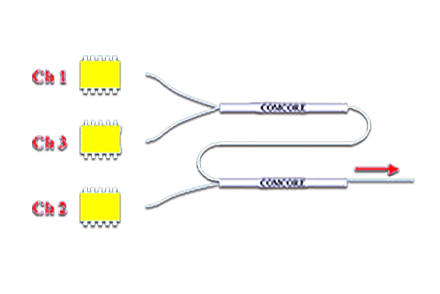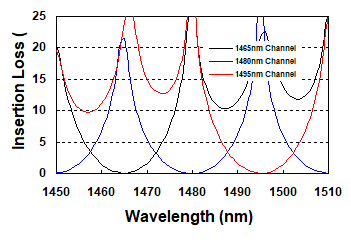### Current position：Home / Products

 14xx Three-Channel Wavelength Pump CombinerProduct Features Product Applications Superfused Allfiber Approach High Power Sources Very Low Insertion Loss Optical Amplifiers Low Polarization Dependent Loss Optical Instrument High Power Handling Ultra-High Reliability
 Parameters Specifications Channel Space nm 10 to 19 20 to 30 Channel Bandwidth nm 2 3 Insertion Loss for Channel 1 and 3* Max dB 0.8 Insertion Loss for Channel 2 Max dB 0.4 Polarization Dependent Loss Max dB 0.2 Isolation Min dB 14 Operating power Max mW 1000 Operating Temperature °C -10 to +70 Storage Temperature °C -40 to +85 Package Type mm S10=Ø3x92: for bare fiber

* Channel1 and 3 are the shortest and the longest wavelength Channels, individually.

 Typical SpectrumOrder information W P C 3 Starting Wavelength 00=1400nm 01=1401nm 02=1402nm … … 50=1450nm 51=1451nm … … 78=1478nm 79=1479nm 80=1480nm Channel Space 10=10nm 11=11nm 12=12nm. … 15=15nm … 19=19nm. 20=20nm … 25=25nm 26=26nm … 29=29nm 30=30nm Package 9=S10 Fiber Type 1=G652 or Equivalent Pigtail S=250μm bare fiber Fiber Length 0=0.5m 1=0.75m 2=1.0m 3=1.5m 4=2.0m S=Specify Connector 0=None 1=FC/PC 3=FC/APC

Note:1. Central Wavelength can be customized for different applications.
2. All specifications are before connectors and are subject to change without notice.